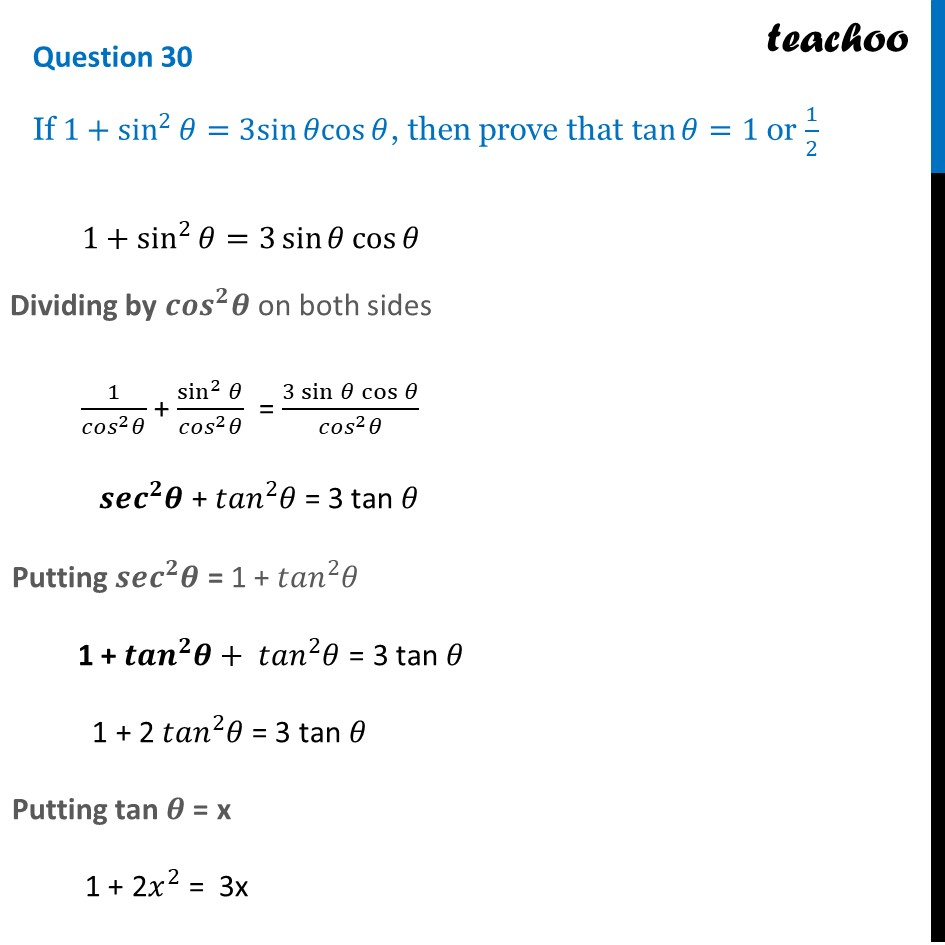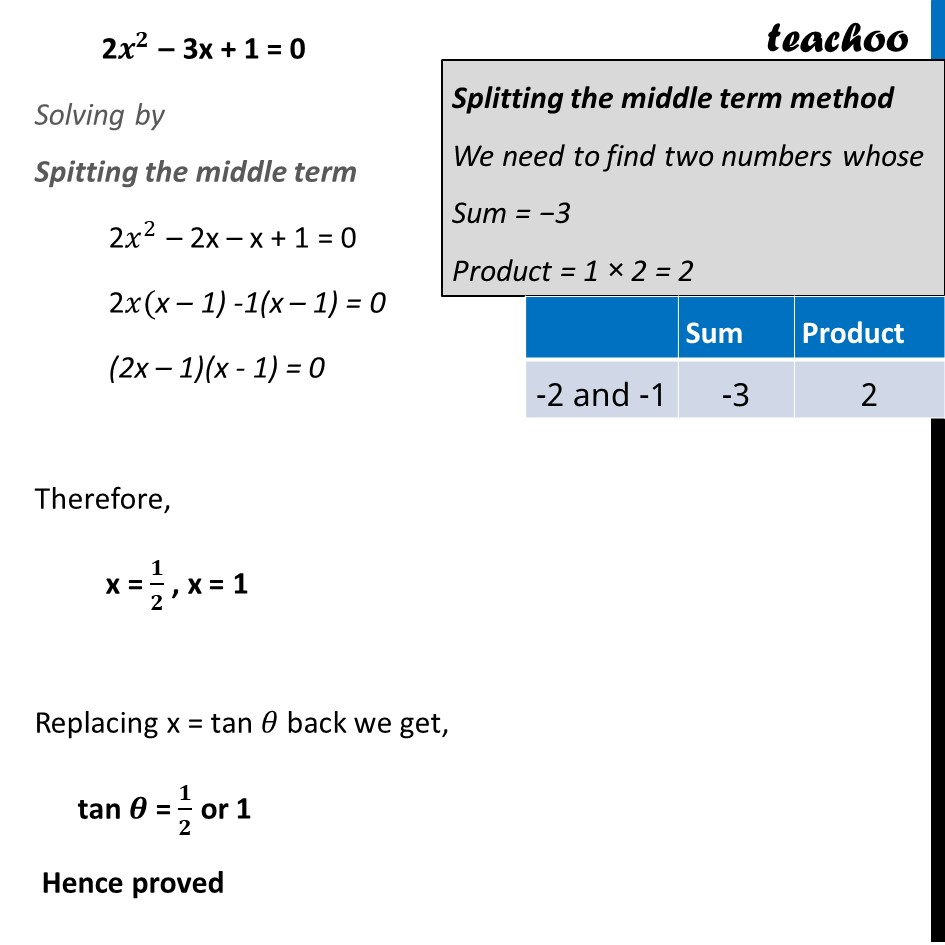CBSE Class 10 Sample Paper for 2024 Boards - Maths Standard

Class 10
Solutions of Sample Papers for Class 10 Boards

## If 1+sin^2⁡θ=3sin⁡θcos⁡θ, then prove that tan⁡θ=1 or 1/2Learn in your speed, with individual attention - Teachoo Maths 1-on-1 Class

### Transcript

1+sin^2⁡𝜃=3 sin⁡〖𝜃 cos⁡𝜃 〗 Dividing by 〖𝒄𝒐𝒔〗^𝟐 𝜽 on both sides 1/(〖𝑐𝑜𝑠〗^2 𝜃) + sin^2⁡𝜃/(〖𝑐𝑜𝑠〗^2 𝜃) = (3 sin⁡〖𝜃 cos⁡𝜃 〗)/(〖𝑐𝑜𝑠〗^2 𝜃) 〖𝒔𝒆𝒄〗^𝟐 𝜽 + 〖𝑡𝑎𝑛〗^2 𝜃 = 3 tan 𝜃 Putting 〖𝒔𝒆𝒄〗^𝟐 𝜽 = 1 + 〖𝑡𝑎𝑛〗^2 𝜃 1 + 〖𝒕𝒂𝒏〗^𝟐 𝜽+ 〖𝑡𝑎𝑛〗^2 𝜃 = 3 tan 𝜃 1 + 2 〖𝑡𝑎𝑛〗^2 𝜃 = 3 tan 𝜃 Putting tan 𝜽 = x 1 + 2𝑥^2 = 3x 2𝒙^𝟐 – 3x + 1 = 0 Solving by Spitting the middle term 2𝑥^2 – 2x – x + 1 = 0 2𝑥(x – 2) -1(x – 2) = 0 (2x – 1)(x - 2) = 0 Therefore, x = 𝟏/𝟐 , x = 2 Replacing x = tan 𝜃 back we get, tan 𝜽 = 𝟏/𝟐 or 2 Hence proved